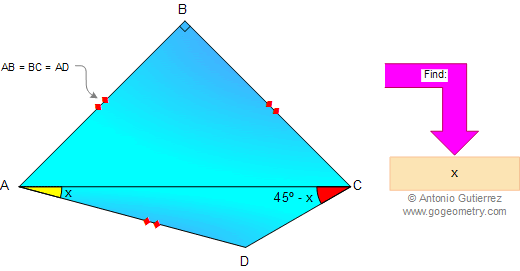## Sunday, May 18, 2008

### Elearn Geometry Problem 8See complete Problem 8 at:
www.gogeometry.com/problem/problem008.htm

Triangle, Angles, Congruence. Level: High School, SAT Prep, College geometry

1.Angle ABC = 90, AB=BC =>ABC is isosceles right triangle => AB=BC=AC/sqrt(2)=>AD=AC/sqrt(2)

Extend DC line past point D. Let AE be a perpendicular that falls to the extension of DC.
Angle ADE = x + (45 - x) = 45. Since ADE is a right triangle, angle DAE=45=>AE=ED=AD/sqrt(2)=AC/2

AEC is a right triangle with AE = AC/2 => angle ACD=30=>x=15

2.after bilding AE perpendicular with CD , a circle
can prescribe ABCE with center O ( midle of AC )
Angle AOE = 2 ACE => angle AEO = EAO = 45 + x =>
AE = r = 1/2 AC => C=30 => x = 15

3.Aplicando la ley de Senos en el triángulo ACD, pues AC=AD*sqrt(2). E·so por cuanto AB=BC=AD. Y listo, y el resto es ejecutar.

4.http://ahmetelmas.files.wordpress.com/2010/05/cozumlu-ornekler.pdf

5.bae deok rak(bdr@korea.com)October 3, 2010 at 5:32 PM

Let E be an intersection of the circumcircle of triangle ABC and the extension line of CD.
Then ang(ADE)45deg and AE=AC/2 and so AED is a triangle with ang(A)=60, ang(E)=90 and ang(C)=30. We get x=15degree.

6.Solution by Cristian

Greetings :)

7.8.http://img9.imageshack.us/img9/3933/problem8.png

We have big angle ∠ (ABC)=360-90=270
Consider circle center B, radius BA=BC
Since ∠ (ADC)=1/2 center angle ∠ (ABC) => D will be on this circle ( see sketch)
And BA=BD=AD => triangle ABD is equilateral

9.In the usual notation c = a = AD and b² = 2a², b = a√2
Denote 45° - x = y
By Sine Rule
a/sin y = AD/sin y = AC/sin 135° = b√2
sin y = a/b√2 = 1/2,
45° - x = y = 30°,
x = 15°

10.draw perpendicular to EC from A,E is point on extension of CE
x=15

11.To Sanoojan Baliah, problem 8.
I can't understand what point is E, defined in the sentence "draw perpendicular do EC from A, E is point on extension of CE".
Could you enlighten it to me?
Thanks.

1.E is on extension of CE,angel AED=90degree

12.To Nilton Lapa

Actually Sanoojan Baliah Says:
Produce CD and Draw a perpendicular to CD from A it is AE.

Greetings and THanks

13.Thanks again for your help. Now I understand the solution presented by Sanoojan Baliah, it is the same idea of Ahmetelmas.

14.Solution- Solucao ( English- Portugues)
English:
I am brazillian so i will try my best to write a solution in english.
Note that angle ACB= angle CAB= 45 deegres
so if we add a angle of X next to angle C we get an 90 degrees angle:
Lets place an triangle identical to ACD, where BC=AD and angle CAD meets angles ACD and ACD to form a right angle. Let J be the vertex of the triangle BCJ , where angles CJB=45-X , JCB= X and CBJ=135 degrees, let JB meet the line AC in P, hence the angle CBP=45 and ABP=45. This provides us with BPC=BPA=90 degrees.
Finally we can assume that AC equal 2L while AB=BC=AD= L times the square root of 2.
The sin law allow us to conclude that :
2L / sin 135 = L time square root of 2/ sin 45- x
Being X< 45 we conclude that
X=15 degrees.

Portugues:
Note que os angulos em A e C sao iguais a 45 graus.
Ainda, percebemos que os angulos em C sao ACB=45 e ACD= 45-X , logo se adicionarmos um angulo X teremos um angulo reto. Posicionando-se um triangulo identico a ADC sobre o lado BC de forma que BC=AD e que o angulo x esteja justaposto a C, temos:
Triangulo construido BCJ = triangulo ADC
assim o angulo CBJ= 135 graus.
Prolongue o segmento JB ate encontrar a reta AC no ponto P, agora note que o angulo CBP=ABJ= 45 graus. Com isso, os angulos APB e CPB sao iguais a 90 graus.
Aplicando do se a Lei do Senos no triangulo ADC obtemos:
2k / seno de 135 graus = k . raiz quadrada de 2 / seno de 45-x
como x< 45
X= 15 graus.
Solution by: Joao Pedro Queroga

15.Bae deok rak(mexcalibur@naver.com)May 20, 2015 at 4:25 PM

Bae deok rak (mexcalibur@naver.com)
angle(ABD)={180-(45+x))/2 and so angle(CBD)=90-(180-(45+x))/2=(45+x)/2.
Therefore we see that 90-x=angle(BCD)=(180-(22.5+x/2))/2, that is, x=15.

16.Extend DB to E such that BE = BA = BC. Then B is the centre of the circumcircle of Tr. AEC. Hence < AEC = 45 ( 1/2 of < ABC = 90). But < ADC = 135 hence this circle passes thro' D too. This implies that Tr. ABD is equilateral from which x+45 = 60 and x = 15.

Sumith Peiris
Moratuwa
Sri Lanka

17.B is the circumcenter of triangle ACD, so BD=AB=AD, triangle ABD is equilateral, <ABD=45+x=60, x=15.

Best regards,
Stan Fulger

18.19.Construct point E such that AE is perpendicular to EC, and triangle ACE is a right-angled triangle with <AEC=90.
Hence <ADE=45, and thus triangle AED is an isosceles right-angled triangle.
Let length of AE be 1.
So AC=2 (Pyth. theorem)
Since AC=2 and AE=1, triangle AEC is a 30-60-90 triangle, with <ACE=30.
Hence, 45-x=30
x=15

20.21.Draw cercle circumscribed to ∆ABC, centered on H, midpoint of AC, since ∆ABC is iscoceles and rectangle at B.
Extend BH to cut circle at E, thus <ABE=45°
Extend CD to cut circle at F.
<ABF=<ACF=45°-x since subtending same arc AF, thus <FBE=x
Draw chord KP//AC. Then <AKP=<CAK=x since they are alternate-internal
Draw chord FL//PK. Then <FLP=<LPK=x since they are alternate-internal
Add the 3 angles: <ABE=45°=<AKP+<PLF+<FBE=3x  x=15°